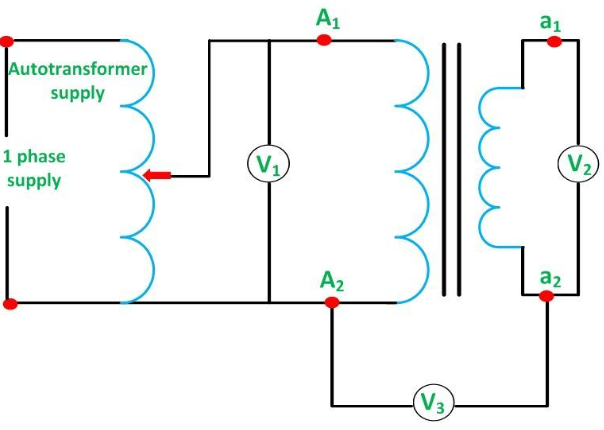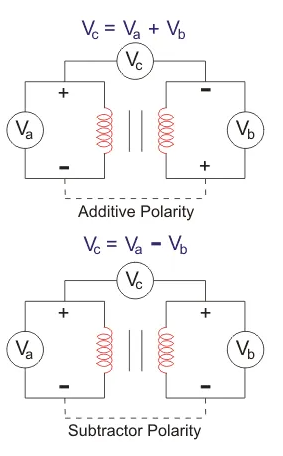# Polarity Test of Transformer

## What is the Polarity Test?

The polarity can be defined as the induced voltage direction in the primary windings and the secondary winding. If two transformers can be connected in parallel, then the polarity must be identified for a good connection of the transformer.

The important role of the polarity test is to make sure that all single-pole devices like switches, circuit breakers, and fuses are allied only in the phase conductor.

## Types of the polarity test

There are two types of the polarity test:In additive polarity, the voltage (Vc) between the secondary side (Vb) and the primary side (Va) will be the sum of both the low voltage and high voltage.

i.e., we will get Vc = Va + Vb

### Subtractive Polarity

In subtractive polarity, the voltage (Vc) between the secondary side (Vb) and the primary side (Va) will be the difference of both the low voltage and high voltage.

i.e., we will get Vc = Va – Vb

In subtractive polarity, if Vc = Va – Vb, it is a step-down transformer, and if Vc = Vb – Va, it is a step-up transformer.

We use subtractive polarity for large-scale transformers and additive polarity for small-scale distribution transformers.

## The procedure of the Polarity Test of the Transformer

1.         Connect the circuit as shown above with a voltmeter (Va) across the primary winding and another voltmeter (Vb) across the secondary winding.

2.         If available, take down the ratings of the transformer and the turn ratio.

3.         We connect a voltmeter (Vc) between secondary and primary windings.

4.         We apply some voltage to the primary side.

5.         By checking the value in the voltmeter (Vc), we can find whether it is additive or subtractive polarity.

If subtractive polarity – Vc should show the difference between Va and Vb.

If additive polarity – Vc should show the sum of Va and Vb.

Caution: the max. measuring the voltage of voltmeter Vc should be greater than the sum of Vb (Secondary winding) and Va (Primary winding) otherwise during the additive polarity, the sum of Vb and Va comes across it.

Note: If we require additive polarity, but we have subtractive polarity, we can simply change it by keeping any of the secondary or primary windings in the same fashion and reversing the winding connection of the other one. Similarly, if we require subtractive polarity but have additive polarity, we could do the same procedure as above.

.(+84) 0913.006.538
Skype, Telegram, Wechat ...